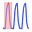#### File Exchange > Data Analysis >    Pulse FitAuthor:
OriginLab Technical Support
8/29/2022
Last Update:
11/4/2022
100
Total Ratings:
0
File Size:
365 KB
Average Rating:File Name:
Pulse_Fit.opx
File Version:
1.00
Minimum Versions:
Type:
App
Summary:

Fit a series of pulses.

Description:

Purpose
This app can be used to find the base markers of a series of pulses and fit pulses using exponential function with growth and decay phases.

Installation

Operation

Activate a worksheet with XY columns or a graph with XY data plots, and then click the app icon to bring up the dialog. Then you can find peaks and base markers in the preview window. After finding the pulses you can choose a fitting function to fit each pulse.

Dialog Settings

• Peak Finding Settings
• Peak Filtering Threshold Height(%): The peaks whose heights are not less than the threshold height will be found.
• Window Points to Find Peaks: Maximum window points to find peaks.
• Base Markers Finding Settings: If auto is checked, use automatic base markers finding settings. Otherwise adjust Estimated Feet Height of Peaks, Maximum Width of Peaks, and Mininmum Width of Peaks to find base markers.
• Fit Control
• Fitting Function: choose ExpGrowDec(Exponential function with one growth and one decay phases), or ExpGrow3Dec2(Exponential function with three growth and two decay phases) to fit a single pulse.
• Maximum Iterate Times: The maximum number allowed iterate times.
• Tolerance: The tolerance value is a stop criterion, default is 1E-9.
• Plot Fit Curve: If checked, fit pulses and plot the fit curve in the preview graph.

Algorithm

$$y=\begin{cases} y_0+A_d+A_g(e^{-x_c/t_g}-e^{-x/t_g}) , & x\leqslant x_c \\ y_0+A_de^{-(x-x_c)/t_d}, &x>x_c \end{cases}$$

$$y=\begin{cases} y_0+A_{d1}+A_{d2}+A_{g1}(e^{-x_c/t_{g1}}-e^{-x/t_{g1}})+A_{g2}(e^{-x_c/t_{g2}}-e^{-x/t_{g2}})+A_{g3}(e^{-x_c/t_{g3}}-e^{-x/t_{g3}}) , & x\leqslant x_c \\ y_0+A_{d1}e^{-(x-x_c)/t_{d1}}+A_{d2}e^{-(x-x_c)/t_{d2}}, &x>x_c \end{cases}$$

Sample OPJU File
This app provides a sample OPJU file.  Right click the App icon in the Apps Gallery window, and choose Show Samples Folder from the short-cut menu. A folder will open. Drag-and-drop the project file PulseFitSampleSample.opju from the folder onto Origin. The Notes window in the project shows detailed steps.
Note: If you wish to save the OPJU after changing, it is recommended that you save to a different folder location (e.g. User Files Folder).